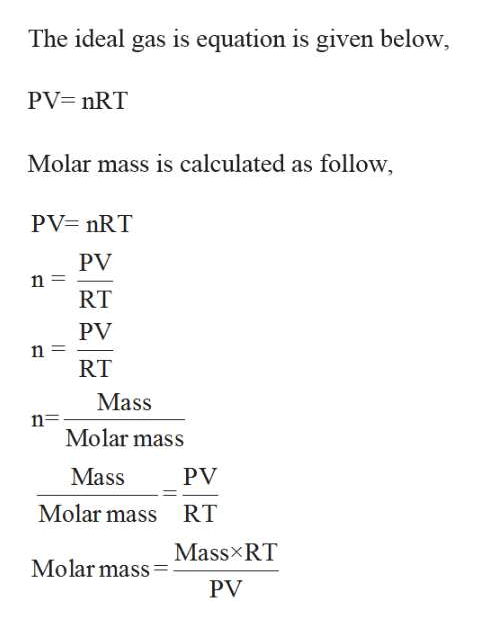# What is the molar mass of a gas if 0.0494 g of the gas occupies a volume of .1 liter at at temperature 26 degrees c and a pressure of 307 torr

Question
76 views

What is the molar mass of a gas if 0.0494 g of the gas occupies a volume of .1 liter at at temperature 26 degrees c and a pressure of 307 torr

check_circle

Step 1

Given,

Mass of the gas = 0.0494 g

Pressure 307 torr,

Volume = 0.1 L

Tempe...help_outlineImage TranscriptioncloseThe ideal gas is equation is given below PV nRT Molar mass is calculated as follow PV nRT PV n RT PV n RT Mass n Molar mass Mass PV Molar mass RT Mass RT Molar mass PV fullscreen

### Want to see the full answer?

See Solution

#### Want to see this answer and more?

Solutions are written by subject experts who are available 24/7. Questions are typically answered within 1 hour.*

See Solution
*Response times may vary by subject and question.
Tagged in

### General Chemistry